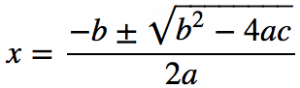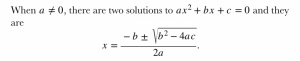# How to add Mathematical Equations in an eBook?

### Why use MathJax?

It can be a tedious task to insert mathematical equations in the eBook. If we create images of each equation and insert them, it disrupts the layout. To avoid this, we can use MathJax.

MathJax allows you to include mathematical equations in your web pages, either using LaTeX, MathML or AsciiMath notation, and the mathematical expressions will be processed using JavaScript to produce HTML, SVG or MathML equations for viewing in any modern browser.

### How to use MathJax?

There are two ways to access MathJax: the easiest way is to use the copy of MathJax available from distributed network service at cdn.mathjax.org, but you can also download and install a copy of MathJax on your own server, or use it locally on your hard disk.

1. Using the MathJax Content Delivery Network (CDN):

To use MathJax from server, you need to do two things:

• Link to MathJax in the web pages that are to include mathematical equations.
• Put mathematical equations into your web pages so that MathJax can display it.

<script type=”text/javascript” async src=”https://cdn.mathjax.org/mathjax/latest/MathJax.js?config=TeX-MML-AM_CHTML”></script>

Consider the following example:

When a≠0, there are two solutions to ax2+bx+c=0 and they areThis is how the expression can be coded using MathJax:

<p>When

<math xmlns=”http://www.w3.org/1998/Math/MathML”>

<mi>a</mi>

<mo>&#x2260;</mo>

<mn>0</mn>

[/itex], there are two solutions to

<math xmlns=”http://www.w3.org/1998/Math/MathML”>

<mi>a</mi>

<msup><mi>x</mi><mn>2</mn></msup>

<mo>+</mo>

<mi>b</mi>

<mi>x</mi>

<mo>+</mo>

<mi>c</mi>

<mo>=</mo>

<mn>0</mn>

[/itex] and they are

<math xmlns=”http://www.w3.org/1998/Math/MathML” display=”block”>

<mi>x</mi>

<mo>=</mo>

<mrow>

<mfrac>

<mrow>

<mo>&#x2212;</mo><mi>b</mi><mo>&#x00B1;</mo>

<msqrt>

<msup><mi>b</mi><mn>2</mn></msup>

<mo>&#x2212;</mo>

<mn>4</mn>

<mi>a</mi>

<mi>c</mi>

</msqrt>

</mrow>

<mrow>

<mn>2</mn><mi>a</mi>

</mrow>

</mfrac>

</mrow>

<mtext>.</mtext>

[/itex]

</p>This method only works when your device has internet connection.

• After that optimize mathjax folder(delete unneccesary files)

<script type=”text/x-mathjax-config”>

MathJax.Hub.Config({

displayAlign: “left”,

jax: [“input/TeX”,”input/MathML”,”output/SVG”],

extensions: [“tex2jax.js”,”mml2jax.js”,”MathEvents.js”],

TeX: {

},

showRenderer: false

},

zoom: “Click”

},

messageStyle: “none”

});

</script>

<script src=”../mathjax/MathJax.js” type=”text/javascript”></script>

Consider the following example:

When a≠0, there are two solutions to ax2+bx+c=0 and they areThis is how the expression can be coded using MathJax:

<p>When

<math xmlns=”http://www.w3.org/1998/Math/MathML”>

<mi>a</mi>

<mo>&#x2260;</mo>

<mn>0</mn>

[/itex], there are two solutions to

<math xmlns=”http://www.w3.org/1998/Math/MathML”>

<mi>a</mi>

<msup><mi>x</mi><mn>2</mn></msup>

<mo>+</mo>

<mi>b</mi>

<mi>x</mi>

<mo>+</mo>

<mi>c</mi>

<mo>=</mo>

<mn>0</mn>

[/itex] and they are

<math xmlns=”http://www.w3.org/1998/Math/MathML” display=”block”>

<mi>x</mi>

<mo>=</mo>

<mrow>

<mfrac>

<mrow>

<mo>&#x2212;</mo>

<mi>b</mi>

<mo>&#x00B1;</mo>

<msqrt>

<msup><mi>b</mi><mn>2</mn></msup>

<mo>&#x2212;</mo>

<mn>4</mn>

<mi>a</mi>

<mi>c</mi>

</msqrt>

</mrow>

<mrow>

<mn>2</mn>

<mi>a</mi>

</mrow>

</mfrac>

</mrow>

<mtext>.</mtext>

[/itex]

</p>

• After Adding MATHML code, close the file and add mathjax folder into OEBPS packages of your file through Total Commander or unzip the file and put mathjax folder in OEBPS Folder.

NOTE: When you reopen the file, remove mathjax folder and after that you can edit it.This is author biographical info, that can be used to tell more about you, your iterests, background and experience. You can change it on Admin > Users > Your Profile > Biographical Info page."

## About us and this blog

We are a digital marketing company with a focus on helping our customers achieve great results across several key areas.

#### Request a free quote

We offer professional SEO services that help websites increase their organic search score drastically in order to compete for the highest rankings even when it comes to highly competitive keywords.

See all posts